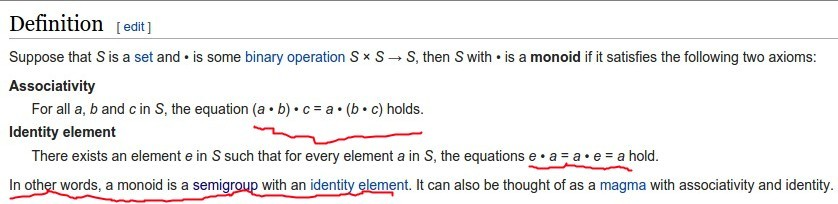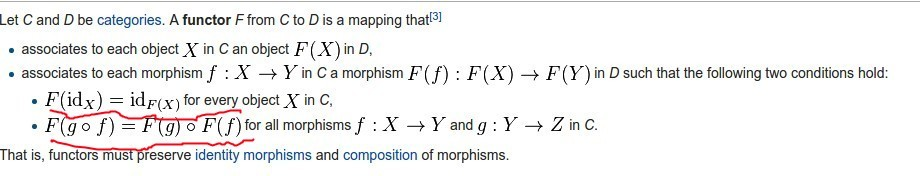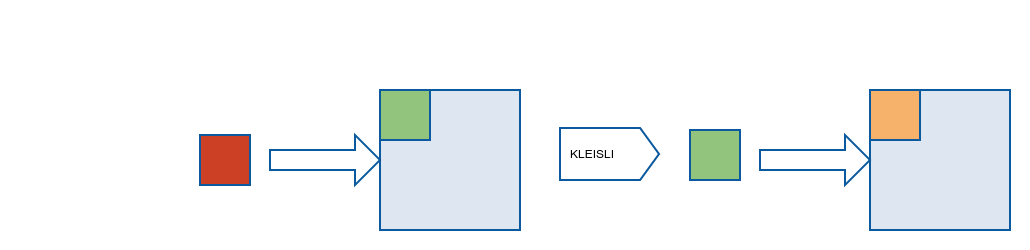# Property Based Testing & FP Abstractions

The Purpose of this workshop is to :

• Learn new testing approach - Property Based Testing.
• Learn practical PBT tool - ScalaCheck.
• Observe some interesting Functional Programming mechanisms and abstractions.
• Gain more motivation to learn FP :)

This approach should expose people to some abstract functional contructs without need to understand every details of their implementation (because we are going to write only tests).

### Exercises

Because participants may have different experience so beside mandatory exercises there are also some additional exercises so when someone will finish mandatory part may start additional part without logging to facebook :)

Examples Can be found here : https://github.com/PawelWlodarski/workshops/tree/master/src/main/scala/jug/lodz/workshops/propertybased

Exercises and Answers can be found here : https://github.com/PawelWlodarski/workshops/tree/master/src/test/scala/jug/lodz/workshops/propertybased

## Start

Imagine you want to test the following functionality :

`````` object MathLib{
//Sum of the first n natural numbers -> SUM=n*(n+1)/2
def sumN(n:Int)=n*(n+1)/2
}
``````

With Unit testing you would write one or couple happy tests and maybe some additional tests for edge cases like 0 or -5. Yet this is far from checking if given rule or property is working properly for all cases.

And this is exactly what we are going to write!

And take a look at the following test :

``````property("for every n>0 where n is Natural SUM(1,2...n)=n*(n+1)/2"){
forAll{(n:Int)=>
println(n) // just for presentation
MathLib.sumN(n) shouldBe ((1 to n).sum)
}
}
``````

After you run it you may see output similar to the following one:

``````-482947512
0
-241473756
0
-120736878
``````

So by default this part :

``````forAll{(n:Int)=>
``````

generates value from whole Integer range and our tests is because we are expecting natural numbers. To change this behaviour we need to create our own Generator

### Generators

First let's try to use default Int generator. To do it we just need to use Arbitrary object

``````Arbitrary.arbitrary[Int]
``````

and set proper constraints in it.

``````Arbitrary.arbitrary[Int].suchThat(i=>i>0)
``````

So now code looks like this :

``````val natural: Gen[Int] =Arbitrary.arbitrary[Int].suchThat(i=>i>0)

property("for every n>0 where n is Natural SUM(1,2...n)=n*(n+1)/2"){
forAll(natural){(n:Int)=>
println(n)
MathLib.sumN(n) shouldBe ((1L to n).sum)
}
}
``````

Notice that forAll now uses our generator. But is our test working now ?

``````Testing started at 18:11 ...
2147483647

TestFailedException was thrown during property evaluation.
Message: -1073741824 was not equal to 2305843008139952128
Occurred when passed generated values (
arg0 = 2147483647
)
``````

Unfortunately our mathematical formula is not prepared for integer overflow which may be the first bug we found in our library but for educational simplicity let's just assume that constraining integer values is also a good solution.

``````val natural: Gen[Int] =Gen.choose(0,1000)

property("for every n>0 where n is Natural SUM(1,2...n)=n*(n+1)/2"){
forAll(natural){(n:Int)=>
println(n)
MathLib.sumN(n) shouldBe ((1L to n).sum)
}
}
``````

And now finally we have a passing test.

Exercise : write test for prepared functions

You have three functions to convert temperature degrees

``````val kelvinToCelsius: Kelvin=>Celsius= k => k - 273.15
val celsiusToFahrenheit:Celsius=>Fahrenheit = c => c * 9/5 + 32.0
val fahrenheitToKelvin :Fahrenheit=>Kelvin = f => (f+459.67) *5/9
``````

Write PBT that is testing property : "(kelvin to celsius to fahrenheit to kelvin) should give first value"

compared values may differ max by +- 0.0001

Also notice how type aliases may be used in scala :

``````type Kelvin = Double
type Celsius = Double
type Fahrenheit = Double
``````

test property : "for every pair of non empty strings (s1.length + s2.length) > s1.length "

## Testing Functions

We know how to test values but how we can test functions and functional abstractions?

To gain knowledge on how to generate functions for property tests first of all we need to learn some new ways of creating Generators.

#### Generators with set of values

Following code will create generator which returns always on of 1,2,5 or 7

``````val simpleGenerator: Gen[Int] =Gen.oneOf(1,3,5,7)
``````

And Another piece of code shows how to use existing generators to combine their results

``````val composedGenerator: Gen[Int] =Gen.oneOf(Gen.choose(0,100),Gen.choose(100,200))
``````

#### Generators Composition

If we have two independent generators we can easily compose their value with simple for comprehension

``````  val pairsGenerator: Gen[(Int, Int)] = for{
i1 <- simpleGenerator
i2 <- composedGenerator
} yield (i1,i2)
``````

And an example usage would look like this

``````property("generators should properly generate pair of integers"){
forAll(pairsGenerator){case (i1,i2) =>
Set(1,3,5,7) should contain(i1)
i2 should (be >= 0 and be <= 200)
}
}
``````

#### Generating functions

Because function is a simple value then we can easily create generator with predefined set of functions

``````val intTointFunctions:Gen[Int=>Int]=Gen.oneOf((x:Int)=>x+1,(x:Int)=>x*2,(x:Int)=>x%10)
val stringToIntFunctions:Gen[String=>Int]=Gen.oneOf((s:String)=>s.toInt,(s:String)=>s.length)
``````

And the usage

``````property("generator should generate a funcion"){
forAll(intTointFunctions){ f:(Int=>Int) =>
f shouldBe a [Function1[_,_]]
}
}
``````

Exercise : write tests functions composition

• implement two Property Tests
`````` //EXERCISES
//MANDATORY
property("for every two functions f1 : String=> Int and f2 : Int=>Int , f1 andThen f2 should be equal to f2(f1(x))"){

}

property("for every two functions f1 : Int=> Int and f2 : String=>Int , f1 compose f2 should be equal to f1(f2(x))"){

}
``````

• prove that Function.curry from standard library works properly
``````  //ADDITIONAL
property("for every f:(Int,Int)=> Int , f.curry should generate equal function f` : Int=>Int=>Int"){

}
``````

# Testing Domain

Till now we were working within mathematical domain so now time for something closer to everyday problems.

Let's define a simple domain :

``````object PBTDomain {
case class Price(value:BigDecimal)
case class Product(name:String, price:Price)
case class Purchase(id:Int,products:List[Product])

trait PurchaseService{
def purchase(purchase:Purchase)(p:Product) : Purchase = Purchase(purchase.id, p :: purchase.products)
}

object PurchaseService extends PurchaseService

}
``````Let's quickly check how to generate a domain object

``````val priceGenerator=for {
i <- Gen.choose(0,Int.MaxValue)
} yield Price(BigDecimal(i))
``````

And again simple for comprehension is enough and usage is really trivial

``````property("price should be larger than 0"){
forAll(priceGenerator){p=>
p.value should be > BigDecimal(0)
}
}
``````

Exercise : write domain generators

We already have generator for price. Now we need two more for product and purchase

``````  //Exercise
property("product should have a name"){

}

property("purchase should not have empty list of products"){

}
``````

Additionally you can write Property Test for the Domain Service

`````` //ADDITIONAL
property("PurchaseService should add product to product list in an existing purchase"){

}
``````

# Testing Functional Abstractions

For this part of the workshop we will import two external libraries

``````libraryDependencies += "org.typelevel" %% "cats" % "0.4.1"
libraryDependencies += "org.scalaz" %% "scalaz-core" % "7.2.1"
``````

Both add additional Functional Programming mechanisms to standard Scala library. The plan is to use Property Based Testing to gain some knowledge and intuition around some interesting FP concepts

## Monoid

According to wikipedia monoid isTo see how this concept can be used in practice take a look at the code in the following file : https://github.com/PawelWlodarski/workshops/blob/master/src/main/scala/jug/lodz/workshops/propertybased/PBTMonoids.scala

We have there examples of two monoids where S can be undertood as Integers but also there is a domain example where S is set of prices

Integers:

``````object IntAddMonoid extends Monoid[Int]{
override def empty: Int = 0
override def combine(x: Int, y: Int): Int = x+y
}
``````

Prices:

``````import PBTDomain.Price

object PriceMonoid extends Monoid[Price] {
override def empty: Price = Price(BigDecimal(0))
override def combine(x: Price, y: Price): Price = Price(x.value+y.value)
}
``````

How to use this mechanism in real code? Below you have small reduce function.

``````def reduce[A](seq:Seq[A])(implicit monoid:Monoid[A]): A ={
seq.fold(monoid.empty)(monoid.combine)
}
``````

Because price monoid is implicit we can treat it as a domain law or property of domain context

In other word it defines what is the domain meaning of reduce in the context of our domain. In this case it will calculate sum of all prices.

``````implicit val monoid=PriceMonoid

reduce(products.map(p=>p.price))
``````

#### cats

In cats library Monoid is a part of algebra package

``````/**
* A monoid is a semigroup with an identity. A monoid is a specialization of a
* semigroup, so its operation must be associative. Additionally,
* `combine(x, empty) == combine(empty, x) == x`. For example, if we have `Monoid[String]`,
* with `combine` as string concatenation, then `empty = ""`.
*/
trait Monoid[@sp(Int, Long, Float, Double) A] extends Any with Semigroup[A] {

/**
* Return the identity element for this monoid.
*/
def empty: A
``````

and it extends Semigroup

``````/**
* A semigroup is any set `A` with an associative operation (`combine`).
*/
trait Semigroup[@sp(Int, Long, Float, Double) A] extends Any with Serializable {

/**
* Associative operation taking which combines two values.
*/
def combine(x: A, y: A): A
``````

Exercise : test monoids

As Mandatory Part write test for both identity and asociativity properties of our second Int Monoid

`````` //EXERCISES
property("IntMultiplyMonoid identity law"){

}

property("IntMultiplyMonoid asociativity law"){

}
``````

Additionally you can write test for PriceMonoid and our library reduce function.

``````  //ADDITIONAL
property("PriceMonoid identity law"){

}

property("PriceMonoid asociativity law"){

}

property("reduce with Price Monoid should count sum of all prices"){

}
``````

## FunctorIn scala List can be treated as a functor and it gives us a possibility to transform List[String] into List[Int] with a function string=>string.length

To gain more intuition let's try to test if List actually respects Functor laws.

• identity
`````` property("List satisfies Functor identity property"){
forAll(notEmptyList){ ls=>
ls.map(identity) shouldBe(identity(ls))
}
}
``````
• composition
`````` property("List satisfies Functor associativity property"){
forAll(notEmptyList,stringToIntFunctions,intTointFunctions){ (ls,fsi,fii)=>
ls.map(fsi andThen fii) shouldBe(ls.map(fsi).map(fii))
}
}
``````

#### cats

To use Functor from Cats in practice we can redefine our reduce method so it adds another abstraction of how collection will be mapped

`````` def reduce[A,B](seq:List[A])(f:A=>B)(implicit monoid:Monoid[B], functor:Functor[List]): B ={
functor.map(seq)(f).fold(monoid.empty)(monoid.combine)
}
``````

Implicit Functor[List] is defined somewhere in cats.std.all._

``````import cats.std.all._
implicit val monoid=PriceMonoid

val products= List(
Product("tv",Price(BigDecimal(300))),
Product("mouse",Price(BigDecimal(20)))
)

println(reduce(products)(p=>p.price))
``````

Exercise : option as a Functor

`````` //EXERCISES
property("Option satisfies Functor identity property"){

}

property("Option satisfies Functor associativity property"){

}
``````

Graphical Explanation of Monad is follwoingSo for example :

• when we are calling database id=>Option[Value] and then we want to use a value to make another call Monad allow us to change result Option[Option[Value]] into Option[Value]
• the same thing can be achieved when we want to call one webservice and then use the result to call another one Future[Future[Int]] -> Future[Int]

With given two simple functions

``````val split:String=>List[String] = s=>s.split(" ").toList
val variants:String=>List[String] = s=>List(s,s.toUpperCase,s.toLowerCase)
``````

In our exercise we are going to test List agains three monad laws

• Left identity
``````property("List satisfies left identity law : M.unit(a).flatMap(f) == f(a)"){
forAll{(s:String)=>
List(s).flatMap(split) shouldBe(split(s))
}
}
``````
• Right identity
``````property("List satisfies right identity law : M.flatMap(M.unit) == M"){
forAll{(ls:List[String])=>
ls.flatMap(a=>List(a)) shouldBe(ls)
}
}
``````
• Associativity
``````property("List satisfies Associativity law : M.flatMap(f1).flatMap(f2) == M.flatMap(x=>f1(x).flatMap(f2))"){
forAll{(ls:List[String])=>
ls.flatMap(split).flatMap(variants) shouldBe(ls.flatMap(string=>split(string).flatMap(variants)))
}
}
``````

Exercise : Option as a Monad

Her we will going to repeat Monad exercise for options and to make it more interesting some constructs from our Purchase Domain will be used.

``````//database
val purchaseWithId7=Purchase(7,List(
Product("tv",Price(BigDecimal(300)))
))
//mock DB
val purchases:Map[Int,Purchase] = Map(7 -> purchaseWithId7)

//curried general function
val findPurchase : Map[Int,Purchase] => Int => Option[Purchase] = m => id => m.get(id)

//specific functions
val findPurchaseInMockDB: (Int) => Option[Purchase] =findPurchase(purchases)
val largest : List[Int] => Option[Int] = l => if(l.isEmpty) None else Some(l.max)
``````

And the exercise

`````` property("Option satisfies left identity law : M.unit(a).flatMap(f) == f(a)"){
//larges
}

property("Option satisfies right identity law : M.flatMap(M.unit) == M"){
//no funcction needed
}

property("Option satisfies Associativity law : M.flatMap(f1).flatMap(f2) == M.flatMap(x=>f1(x).flatMap(f2))"){
//use largest and find purchase in mock DB.
}
``````

What if for example we have two effects at the same time? Foe example when we are calling external webservice we have time - Future[T] and posibility that given value will be missing - Option

In this situation we can use predefined MonadTransformer . In Graphical explanation it will look like this :Let's imagine that in the code we have two web services :

`````` def service1(name:String) : Future[Option[Int]] ...
def service2(id:Int) : Future[Option[Company]]
``````

We can not write simple for comprehension because argument of second web service Int is not the same as result of the first one Option[Int].

``````  import cats.data.OptionT
import cats.std.future._

val result=for{
id<-OptionT(service1("company1"))
company<-OptionT(service2(id))
} yield company.info

val finalValue: Future[Option[String]] = result.value
``````

More here -> http://typelevel.org/cats/tut/optiont.html

## Kleisli

Kleisli allow us to connect two so called monadic functions A=>M[B] and B=>M[C]So a very quick example would be :

``````  import cats.data.Kleisli
import cats.std.all._
val split:String=>List[String] = s=>s.split(" ").toList
val variants:String=>List[String] = s=>List(s,s.toUpperCase,s.toLowerCase)

val variantsK = Kleisli(split).andThen(variants)

println(variantsK.run("this is a sentence"))
//List(this, THIS, this, is, IS, is, a, A, a, sentence, SENTENCE, sentence)
``````

And this was the last example from this workshop! Remember what was educational purpose of this material

• Learn how to use Scalacheck
• Gain better intuition around concept "Function as a Value"
• Take quick glance at some very interesting Functional abstractions

# Homework

• You can check Basic Scalacheck course :https://pawelwlodarski.gitbooks.io/workshops/content/scalacheck.html
• Try to finish every exercise from this workshop on your own. Play with examples and test additional possibilities of Scalacheck
• Prove that According to Amdahl Law : S(n) = T(1) / T(n) = 1 / (B + (1 – B) / n) where
• n - number of available threads
• B- part of program which is sequential
• then if B = 5% , you will receive max 20 time speedup when you have 1000 cores!!!